edHelper subscribers - Create a new printable

Sample edHelper.com - LinearEquations Worksheet

 Name _____________________________Date ___________________
Linear Equations
Match the equation with its graph.
 3x + y = 21 x + 7y = 48 x + 2y = 10
 5x + 3y = 30 x + 5y = 51 6x + 7y = 77
 x + 2y = 1 2x - 7y = 49 6x - 7y = 7

 1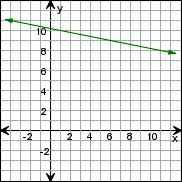2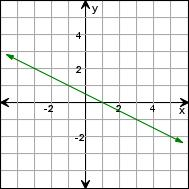3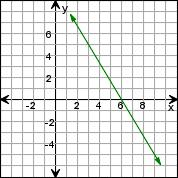4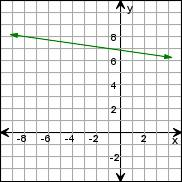5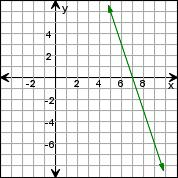6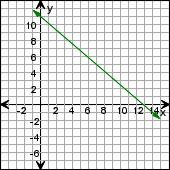7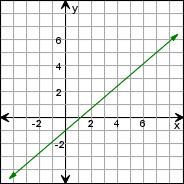8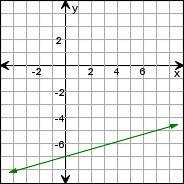9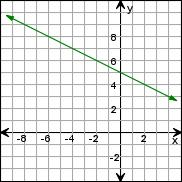Sample
This is only a sample worksheet.

edHelper subscribers - Create a new printable